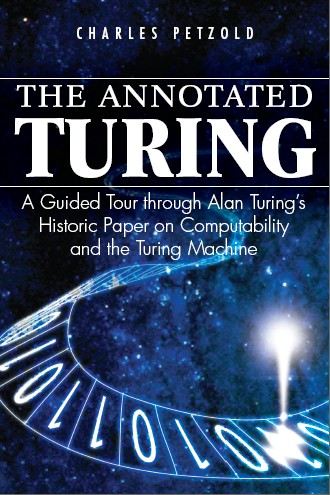# The Annotated Turing## Chapter 2 - The Irrational and the Trascendental

There's one thing in chapter 2 (page 31) I find hardly convincing.

Petzold is talking about the Continuum Hypothesis, formulated (but not proven) by Cantor.

$\aleph_1 = 2^{\aleph_0}$

Before that, he shows that $$|\mathbb{R}| = 2^{\aleph_0}$$.

The general rule is that:

$\textrm{cardinality of a power set} = 2^{\textrm{cardinality of the original set}}$

Here what happens if we construct the power set of the natural numbers.

We must find some order in our operations. The infinite sequence of natural numbers is set as a header. Then, for each row, we put 0s or 1s depending on whether the corresponding number is in the current element of the power set.

0 1 2 3 4 5 Subset
0 0 0 0 0 0 $$\{\}$$
1 0 0 0 0 0 $$\{0\}$$
0 1 0 0 0 0 $$\{1\}$$
1 1 0 0 0 0 $$\{0,1\}$$
0 0 1 0 0 0 $$\{2\}$$

And so on. Proceeding like that we construct every possible infinite sequence of 0s and 1s, each one corresponding to one element of the powerset of $$\mathbb{N}$$.

If these sequences of symbols are interpreted as binary encodings of real numbers (like $$100000… \rightarrow .100000…$$), then they are all the real numbers between 0 and 1.

Since this set can be put in correspondence with the entire set of real numbers, it follows that the cardinality of $$\mathbb{R}$$ and the cardinality of the powerset of $$\mathbb{N}$$ are the same.

In a footnote, Petzold says that one must not believe that we found a way to enumerate all reals (which is indeed impossible), because there are no transcendental numbers in the set. That is true, Petzold says, because "each number has a finite number of 1s after the period".

I'm sure that is true, but didn't we just say that we listed all real numbers? Isn't "all real numbers between 0 and 1 except for the transcendental numbers" a different set?

This is what I find not very convincing.

Date: 2016-04-18 Mon 00:00

Created: 2020-10-13 Tue 09:13

Validate